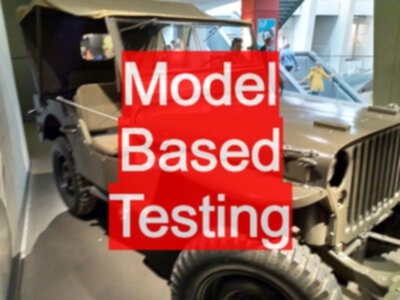# Model Based Testing

Feb 13, 2022

TLDR; Given a model, we can apply a technique. Given a model we can apply many techniques. Techniques are a way of extracting a coverage model from an input model

## Learn Modelling, Techniques Follow

Software testing techniques are model based, not white, Gray, or black box based. In fact, most software techniques are graph based. One reason why people don’t like formal software testing techniques is that the focus is on the technique and not the input model.

All formal techniques require an input model. Many techniques use the same input model.

So rather than learn lots of techniques, you learn a couple of models and then figure out what techniques can apply. And even if you don’t know any techniques, the model itself will help you test.

People face the issue that they’re trying to work out what techniques are appropriate when that is the wrong place to start.

Work out what models are appropriate for your situation, then they can become input models to techniques.

And one of the secrets is that most of the models are graph models. Circles and lines, flows.

Pretty much every white box technique listed is actually a graph model based technique. They’re not source code based, they are graph based. But the input to the creation of that graph model was source code.

The graph is the key to the technique, not the source code.

I looked on Wikipedia at Blackbox Software Testing Techniques and then have a look at Boundary Value Analysis and the example on the Wikipedia page was illustrated with a source code snippet because it’s black box.

So you explain it with code, but then that would make it white box. But if all code is a graph, and we’re really saying that one input to Boundary Value Analysis is a graph where the edges have transition conditions, kind of like flow charts, which could also be used as an input to Boundary Value Analysis because it’s not the flow that feeds into Boundary Value Analysis, it’s the combined set of data ranges across the transitions in that flow.

We have to understand the model that feeds into the technique. All we are taking from that graph model on Wikipedia are the transition conditions, and they could be represented as part of a requirement model, or possibly a truth table, or possibly a set of acceptance criteria, or possibly a set of ordered sets.

There’s lots of ways of creating the boundary lists that feed into Boundary Value Analysis, and it’s important to understand the models that feed into the techniques.

They’re not white or Gray or Black. Techniques are fed an input model.

Most people don’t even think of the input model as a separate thing, but the modeling activity may well be the most important activity. The application of the technique is basically just a coverage technique.

Techniques are a formal way of attaining coverage, or a coverage list, from some input model. And input models can be generated from different sources.

Once you learn that, software techniques become a lot easier to understand.

This was originally recorded on Racket in 202106